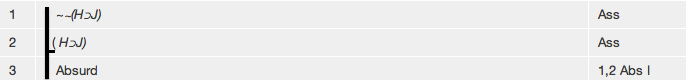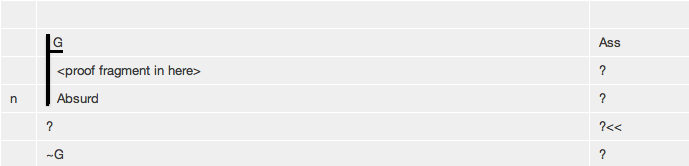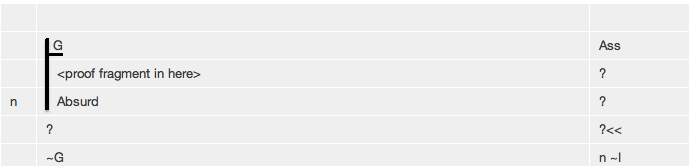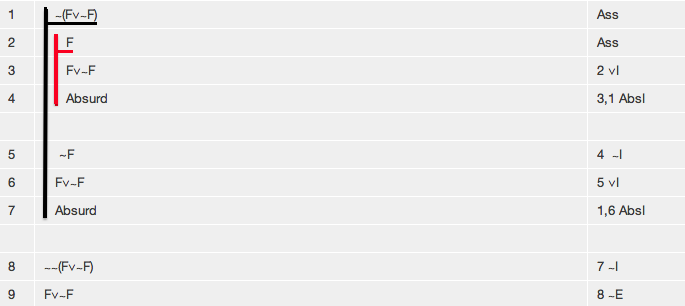# Tutorial 8: Reductio ad Absurdum

Logical System

2013

### Skills to be acquired:

Learning reductio proof, both as plain Negation Introduction and via (double) Negation Elimination (to prove some formulas that do not have negation as their main connective).

### The Tutorial:

Reductio ad Absurdum is the second of the classical forms of inference.

The idea of it is the following. In a valid argument the truth of the premises guarantees the truth of the conclusion or, to put it another way, the falsity of the conclusion guarantees the falsity of at least one of the premises. Some formulas are sure to be false; contradictions, for example, like (A ∧∼A). So, if you can derive a contradiction from just one premise, that proves the premise to be false. But proving that a statement is false is exactly the same as proving that the negation of that statement is true. To sum up, one way of proving the negation of a statement is to assume the statement and derive a contradiction from that assumption. This process is known as Reductio ad Absurdum, and one early example of its used was in the discovery of irrational numbers back in Greek times (the mathematical theorem 'It is not the case that there are two natural numbers such that one divided by the other equals the square root of two' was proved by assuming 'There are two natural numbers such that one divided by the other equals the square root of two' and deducing a contradiction from this assumption).

In our rule system Reductio ad Absurdum appears as the rule for the Introduction of Negation ∼I. This, then, is a rule which gets used when you are trying to obtain a formula which is a negation.

∼I is implemented to two slightly different ways, and you can take your choice among them. The first uses the propositional constant Absurd -- this implementation is easier to understand, makes simple derivations using ∼I one line longer, can make more difficult derivations using ∼I many lines shorter, and fits better with the use of Tactics. It is the recommended implementation for beginners. The other will be described briefly later.

As a preliminary, let us look at another Rule, the one for the Introduction of Absurdity. Our logical system contains the propositional constant Absurd which may be derived from any pair of formulas one of which is the negation of the other; in other words, the pair of formulas must contradict each other and if they do Absurd may be derived. The Introduction of Absurdity is just like the And Introduction except that the conjuncts must contradict each other. Here are some proof fragments illustrating the introduction of Absurd.Notice that the formulas that lead to Absurd contradict each other. Returning to Reductio ad Absurdum and the Rule for the Introduction of Negation...the circumstance that Introduction of Negation gets invoked looks like this.

 ? ? << ~G ?

That is, the formula you are trying to obtain has a ∼ as its main connective-- the formula you are trying to obtain has the form ∼<formula>. In the illustration, for example, G is the <formula>.

You follow a three stage process. First you assume the <formula>. The illustrated fragment will then become

 1 G Ass 2 ? ? << 3 ~G ?

Second you continue deriving in this new context until you manage to derive Absurd thusFinally you select Absurd and click ∼I to complete the Reductio proof.(Remarks for experienced logicians. The second implementation of ∼I is similar except that you omit the step for introducing Absurd-- merely by adding an assumption, eventually selecting two formulas, one of which being the negation of the other, and clicking ∼I, will add the negation of the assumption. Use this if you wish to. On the face of it this method might seem better, but it runs into awkwardnesses when combined with other rules that involve subproofs-- experts might care to think over derivations like F∨G,∼F,∼G ∴ ∼H or (∀x)∼Fx∴∼(∃x)Fx. Part of the problem is that this style of Reductio uses two lines, whereas subproofs are exited with one line, so contrivances are needed.)

There is a second technique based on Reductio and ∼I, and it can be used to prove formulas which do not have a negation as their main connective. Consider this, admittedly advanced, proof of ∴ F∨∼F,Notice lines 8 and 9. Line 8 has been obtained by Negation Introduction (or Reductio), but then its double negation has been taken out by Negation Elimination to give Line 9.

So, briefly put, if you embark on a Reductio, you assume the 'opposite' of what you want to prove. If you want to prove ∼<formula> you assume <formula>. If you want to prove <formula> you assume ∼<formula> (but in this case you go via the double negation, which you eliminate with Negation Elimination).

## Exercises to accompany Tutorial 8

### Exercise 1 (of 4)

There is a Rule for Absurdity Introduction-- in effect it is just And Introduction, but with the requirement that the conjuncts contradict each other. To use it you select a formula, and select the negation of that formula, and choose Absurd I.

Derive the following, either with or without Tactics. (Tactics will ask to you choose where the contradiction will come from; but it also gives you a hint as to the possibilities by listing all potential positive conjuncts.)

a) F, ∼F ∴ Absurd
b) F, F⊃∼F ∴ Absurd
c) F,G,∼(F∧G) ∴ Absurd
d) F, ∼(F⊃F) ∴ Absurd

### Exercise 2 (of 4)

Derive the following. First use Tactics. Start the derivation, switch on Tactics under the Wizard, then click ∼I to lay out the derivation properly. Then repeat the derivations without using Tactics.

a) F ∴ ∼(∼F)
b) F ∴ ∼(∼(∼(∼F))) (*Tactics give you a poor hint here*)
c) ∼F ∴ ∼(F∧G)
d) F∧∼G ∴ ∼(F⊃G)
e) F∧∼G ∴ ∼(F≡G)
f) ∴∼(F∧(∼F))
g) C∧(∼F), A∧((∼G)∧B) ∴ ∼(F∧G)

### Exercise 3 (of 4)

Derive the following. There is a circumstance in which Reductio is used where the initial target formula in not a negation-- the derivation proceeds by assuming a negation using Reductio to get a double negation, then using (double) Negation Elimination to get the desired formula. The first derivation that follows is an illustration of this.

Use Tactics if you wish. (Start the Proof, Switch on Tactics, Choose ∼E, Choose ∼I. That will lay the proof out correctly.)

a) F∧(∼F) ∴ G
b) ∼(F∧G),F,G∴H
c) ∴ F∨∼F (*this is difficult, but important-- learn how to do it*)
d) ∼(F∧G)∴∼F∨∼G

### Exercise 4 (of 4)

There are a number of important theorems which can be expressed as equivalences-- here we consider slightly weakened versions of them which are conditionals.

Derive

Double Negation

a) ∴ F⊃∼∼F

Transposition

b) ∴ (F⊃G)⊃(∼G⊃∼F)
c) ∴(∼G⊃∼F)⊃(F⊃G) (*The automatic theorem prover gives a very long winded proof of this due to missing the trick about using reductio to prove the double negation of what you want.*)

De Morgan's Laws

d) ∴ ∼(F∧G)⊃(∼F∨∼G)
e) ∴ ∼(F∨G)⊃(∼F∧∼G)

Implication

f) ∴ (F⊃G)⊃(∼F∨G)